As already explained, in an extrinsic semiconductor the number of holes is equal to the number of free electrons. Due to thermal agitation, new electron-hole pairs are continually generated while other electron-hole pairs disappear as a result of recombination i.e., free electrons falling into empty covalent bonds. On an average, a hole exists for τh second known as mean lifetime of hole and similarly an electron exists for τe second known as mean lifetime of electron. These Carrier Lifetime in Semiconductor vary from few nanoseconds to several microseconds. The mean Carrier Lifetime in Semiconductor (holes and electrons) form very important factors in semiconductor devices because they indicate the time required for hole and electron concentrations to return to their equilibrium values after being influenced by any external cause such as illumination by light.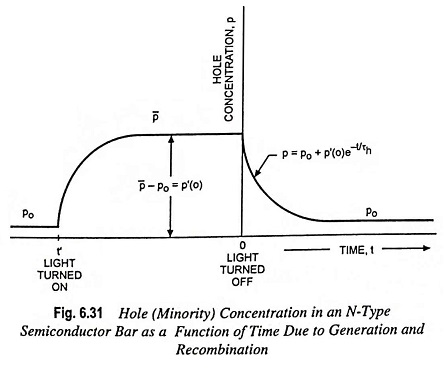Let us consider an N-type silicon bar containing the thermal-equilibrium concentration p0 and n0 of holes and electrons respectively. Assume that at any instant t = t’ the bar is illuminated (Fig. 6.31) and that additional EHPs are produced uniformly throughout the crystal. An equilibrium condition is attained, and the new concentrations are p̅ and n̅ under the influence of radiation. The photo-injected, or excess, concentration is p̅ – p0 for holes and is n̅ – n0, for electrons. As EHPs (electron-hole pairs) are generated due to radiation, then clearly,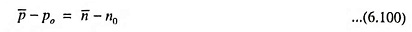Although the increases in hole concentration and electron density are equal, the percentage increase for electrons in an N-type semiconductor is very small while percentage increase in holes may be tremendous. This is because electrons are plentiful and holes are scarce in an N-type crystal. In short, it can be said that the majority carrier concentration is hardly affected due to radiation. So our discussion will be limited to the behavior of the minority carriers.

When a steady-state condition is reached, at time t = 0 (Fig. 6.31), the radiation is removed. Now it is to be shown that the excess carrier density returns to zero exponentially with time.

From the definition of mean lifetime τh and assuming that τh is independent of magnitude of the hole concentration,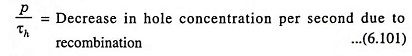From the definition of generation rate,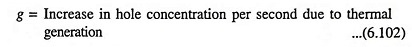Since charge can neither be generated nor destroyed, the increase in hole concentration per second must be equal to dp/dt. This rate must, at every instant of time, equal the algebraic sum of the rates given in Eqs. (6.101) and (6.102) i.e.,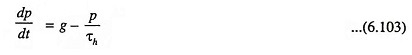Under steady-state conditions, the rate of increase in hole concentration dp/dt must be equal to zero, and with no radiation falling on the sample, the hole concentration attains its thermal-equilibrium value p0.

So g = p0h and the above Eq. (6.103) becomes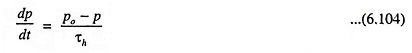The excess, or injected, carrier density p’ may be defined as the increase in minority concentration above the equilibrium magnitude. Since p’ is a function of time, then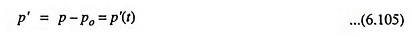From Eq. (6.104), the differential equation controlling p’ is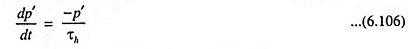The rate of change of excess concentration is proportional to this concentration—an intuitively correct result. The minus sign indicates that the change is a decrease in the case of recombination and an increase when the concentration is recovering from a temporary depletion.

Since the radiation causes an initial (at ≤ 0) excess concentration p′(0) = p̅ – p0 and then this excitation is removed, the solution of Eq. (6.106) for t ≥ 0 is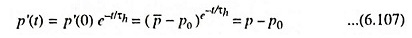The excess concentration falls exponentially to zero (p’ = 0 or p = p0) with a time constant equal to the mean lifetime τh, as shown in Fig. 6.31.

The most important mechanism in silicon or germanium through which recombination of holes and electrons occur is the mechanism involving recombination centres or traps which contribute electronic states in the energy gap of the semiconductor material. These new states are associated with imperfections in the crystal. Specifically, metallic impurities in the semiconductor are capable of introducing energy states in the forbidden gap. Recombination is affected not only by volume impurities but also by surface imperfections in the crystal.

Gold is extensively employed as a recombination agent in semiconductor manufacture. Thus the designer of the semiconductor device can obtain desired Carrier Lifetime in Semiconductor by introducing gold into silicon under controlled conditions.

Scroll to Top